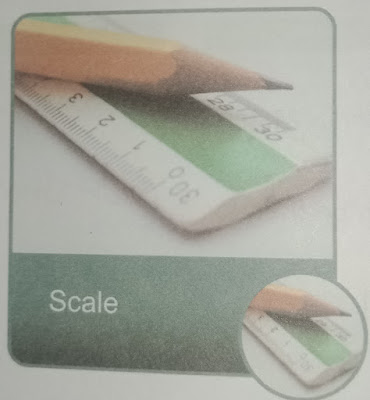# Addition and Subtraction of Units of Lengths

Lesson Planning of Addition and Subtraction of Units of Lengths

Subject Mathematics

Students` Learning Outcomes

• Add and subtract expressions involving similar units of lengths.
• Use appropriate units to measure lengths of different objects.
• Solve real life problems involving conversions, addition and subtraction of units of lengths.
• Information for Teachers
• Milometer, meter, centimeter and millimetre are units of measurement written as km, m, cm and mm respectively.
• Conversion of units can be done by using the following rules, as;

1 KM = 1000 M

1 M = 10 CM

1 CM = 10 MM

• With the help of these units of length we can find required measurements and can also change them into appropriate measure.
• We multiply while converting greater to smaller unit and use division while converting small unit to greater.
• During this lesson consult text book at each lever wherein it is required

Material / Resources

Writing board, chalk/marker, scale, measuring tape, textbook

Introduction

• Write on the board kilometres, meters, centimetres and millimetres.
• Ask the students which unit can be used for measuring the following: as;
• Distance between cities (Milometers)
• Length of a pencil (Centimetre)
• The thickness of finger nails (Millimetres)
• Tell them that we have learnt basic units of length in previous lectures.
• Today we will learn how to add and subtract these units.
• In fact, only similar units can be added or subtracted
• We make different units similar before adding and subtracting.

Development

 Activity 1 Ask the class how many units can you see written on a scale? (Expected answer would be as; inch, centimeter and millimetre) Observe how many centimetres does it contain? (Expected answer would be as; 30 cm) If I have 4 such scale how many centimetres they will make altogether? 1 Scale = 30 cm 4 Scale = 30 cm + 30 cm + 30 cm = 120 cm.Activity 2 “Zenith went to a tailor and gives him 4 meters cloth to stitch her shirt. Tailor returned 1 meter cloth to her after stitching shirt. How much cloth is used in her shirt?” Solve on the board with the help of subtraction.Ask them do you all wear same size of uniform? (Expected answer would be as; No) Tell them that usually the distance is measured in kilometres, whereas the length are measured in meters and centimetres and even very small lengths are measured in millimetres. Give examples: as; Distance from Lahore to Karachi = km The length of a Bundle of cloth = m The length of a needle to stitches – mm Call the name of different things from the classroom and ask the students at random which unit they will use to measure each one of them.

Sum up / Conclusion
• Same units can be added or subtracted only.
• If we want to add them we first convert them in same units.
• The smallest unit for measuring length is millimetre.
• The greatest unit for measuring length, height or distance is kilometers.
• We multiply while converting greater to smaller unit and use division when we convert smaller to greater unit.

Assessment

• Give following problems to the students to assess them: as;
• Distance between cities A to B is 450 kilometres. If the driver has covered 291 kilometres, then how many kilometres are left to be covered?
•

• Choose the appropriate units for measuring the following lengths: as;
• Length of shoes:

(a                   (a) Meter  (b) centimetres  (c) millimetres

• Width of an eraser:

(a                 (a)  Millimetre  (b) meters   (c) kilometres

• Assign following questions to the students: as;

• Choose the best estimate to answer each question: as;
• About how tall is a plastic water bottle?

(a) 20 cm   (b)  20 km

© 20 m    (d) 20 mm

• About how long a pens nib is?

(a                        (a)   2 cm   (b)  2 mm

(c)  2 m     (d) 2 km

• About how away from can be my school from my home?

(a                           (a) 1 m    (b)  1 km